## STAM 102 :: Lecture 11 :: t-test for two samples and ANOVA with One-way classification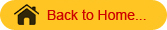# t-test for two samples assuming equal variances:

• Example: Perform t-test for two samples assuming equal variances for yield of food in kg/ha in Kharif  and Rabi season from 1996-97 to 2006-07.
• The data is entered in Excel sheet as shown below: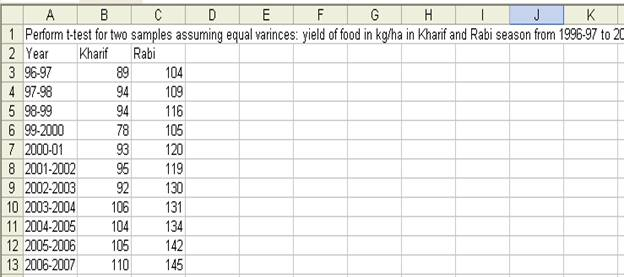• Choose t-Test: Two-Samples assuming Equal Variances in the Data Analysis window: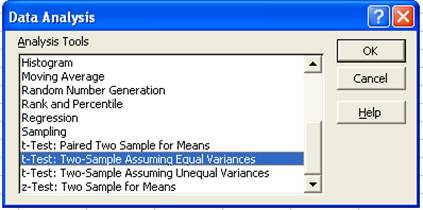• In the t-Test: Two-Samples assuming Equal Variances window enter the Variable 1 Range and Variable 2 Range.
• Check the Labels option
• Hypothesized mean difference be 0.5
• Let Alpha value be 0.05
• Set output range as E2.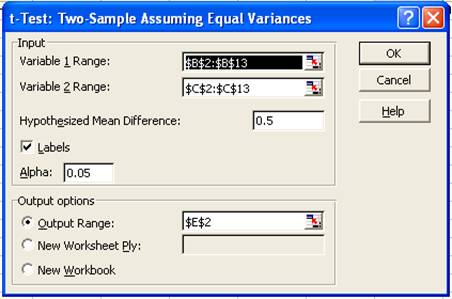• The result will be displayed from E2 as shown below: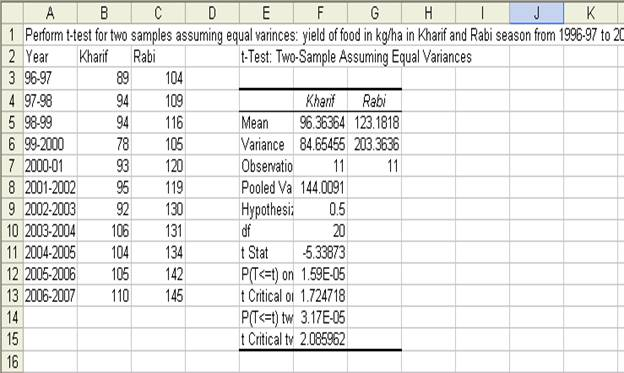ANOVA(Analysis od Variances) with One-way Classification

• Example: Perform ANOVA One Way Classification for yield of food in kg/ha in three seasons from 1996-97 to 2006-07
• The data is entered in Excel sheet as follows: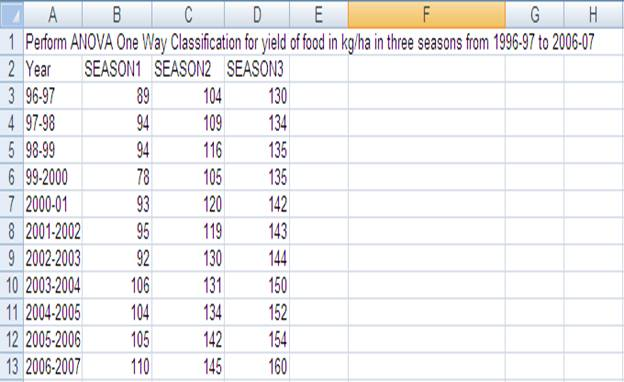• Choose ANOVA: Single Factor from Data Analysis window.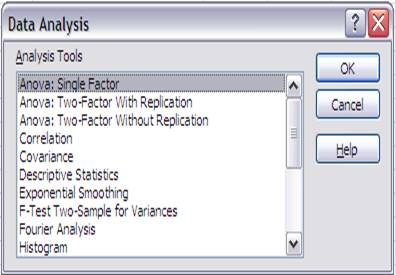• In the ANOVA: Single Factor window enter the input range. The input range in the example is B2:D13
• Choose Group by Columns
• Check Labels in the first row
• Output range is set to F2 as follows: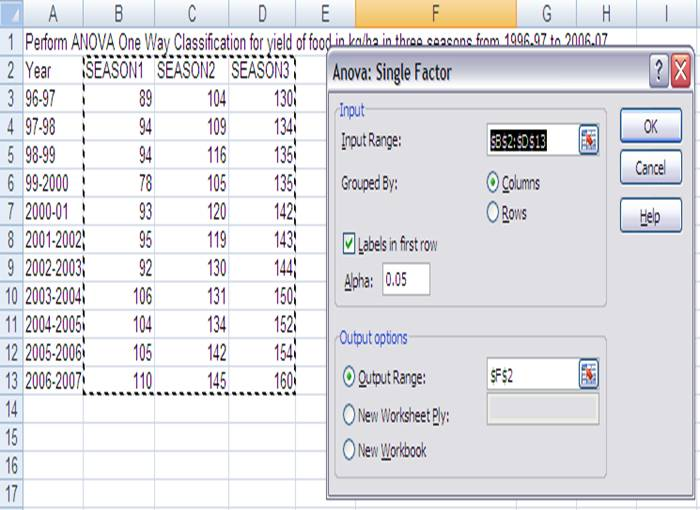• The result of the ANOVA with One-way classification is displayed from F2 as shown below: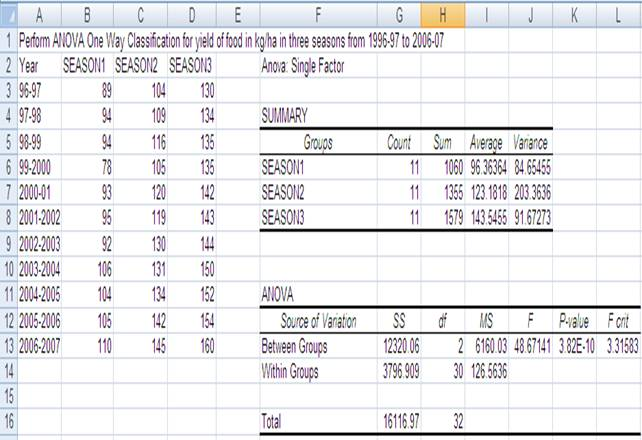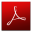Download this lecture as PDF here